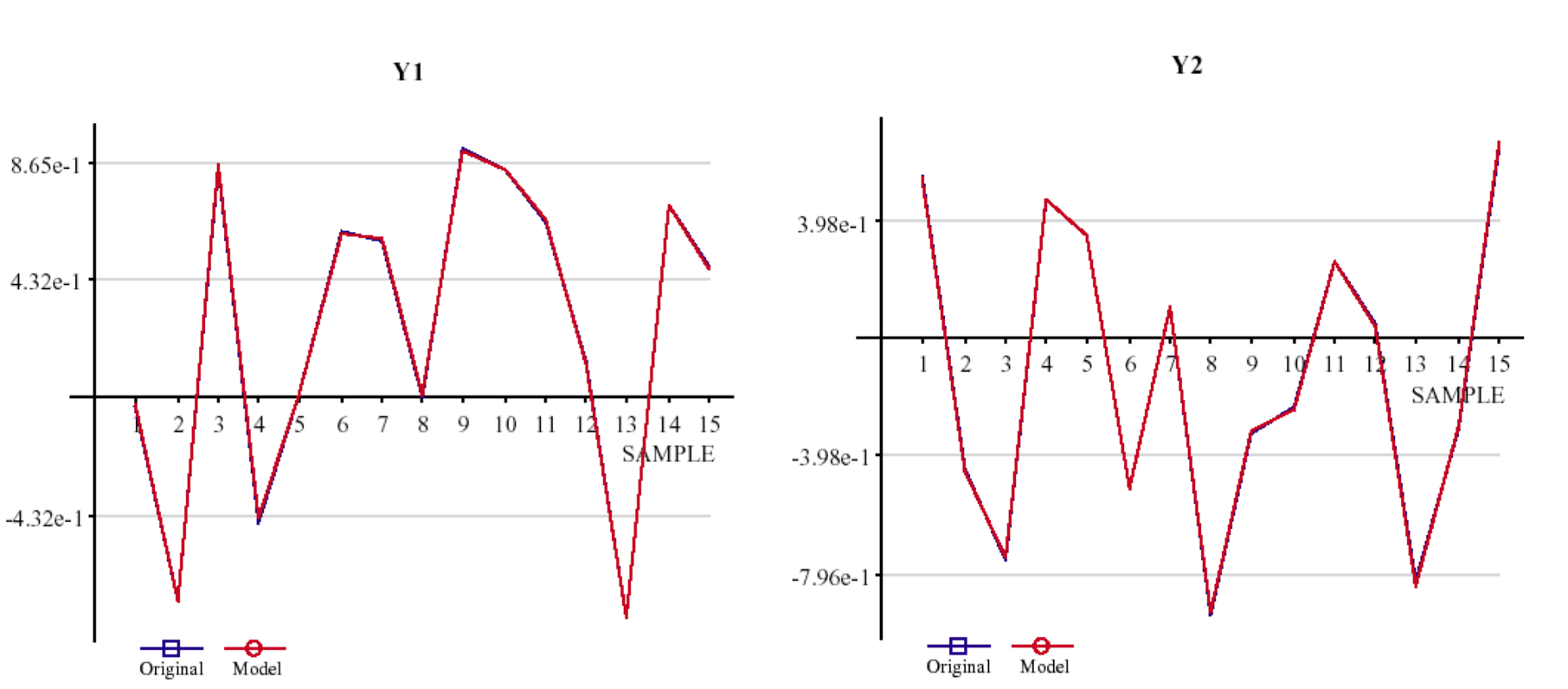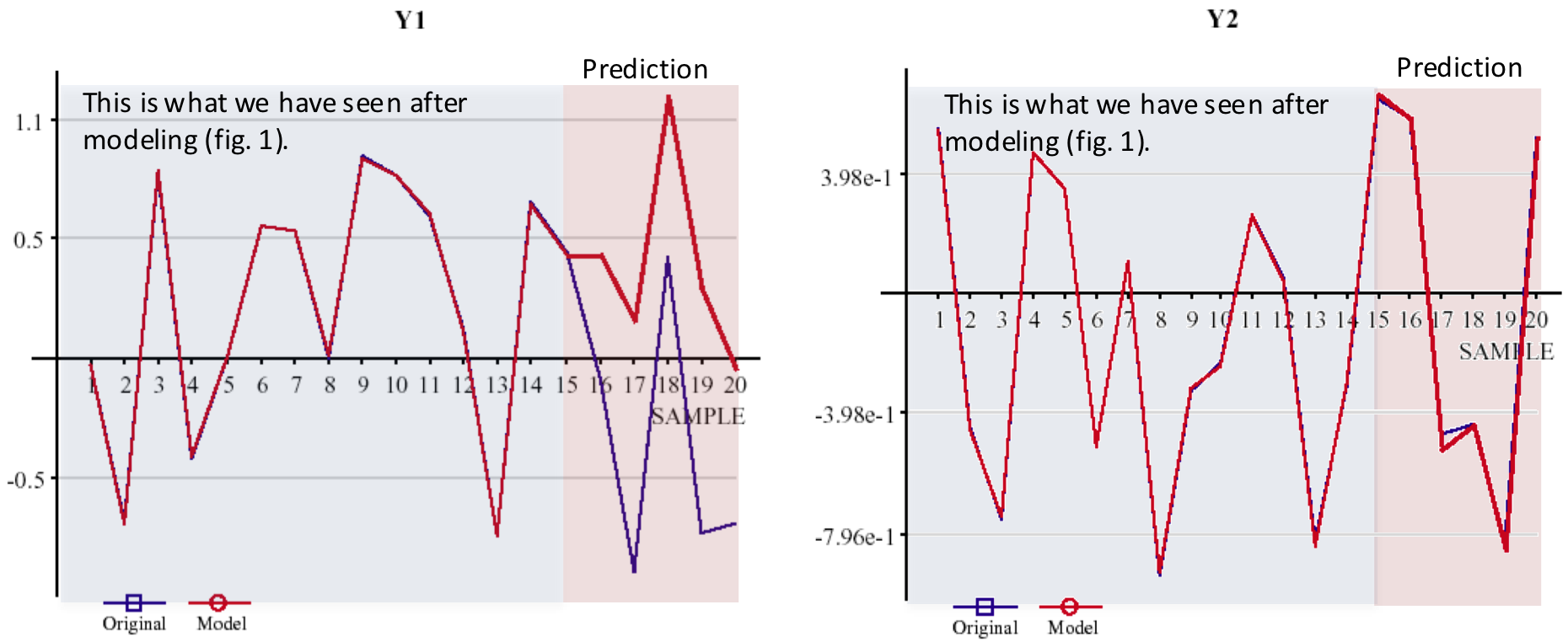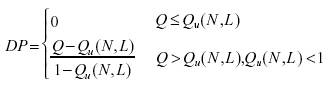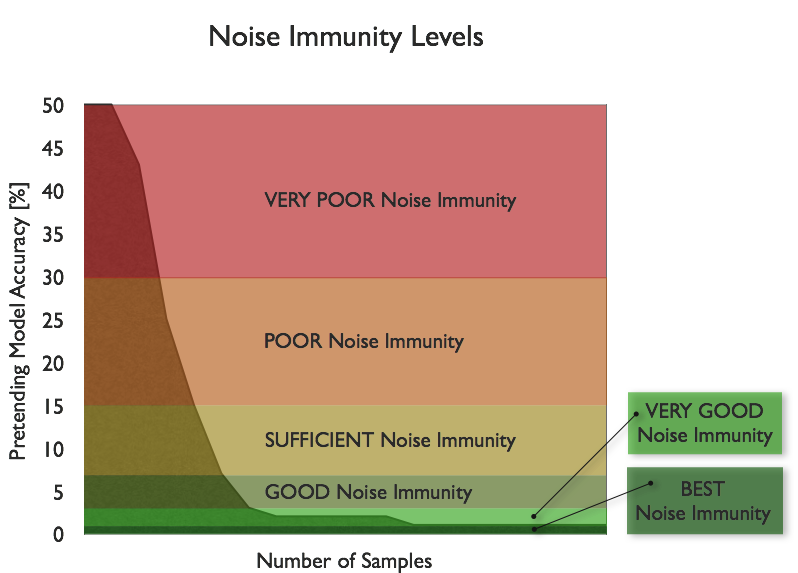INSIGHTSMulti-level Model Validation. Innovation for reliable predictive models.
 One original and powerful feature implemented in Insights is additional external evaluation of self-organized linear and nonlinear analytic models. This document is about to show how this new model evaluation approach actively supports answering the question if the obtained model reflects a valid, reliable, and in best case, causal relationship or if it's just pretending model accuracy due to chance correlation, only. Also, a new model quality measure that takes into consideration the noise filtering power of the modeling algorithm and model complexity is introduced: Descriptive Power. The Problem A key problem in data mining is final evaluation of developed models. This evaluation process is an important condition for deployment of data mining models. By learning from a finite set of data, only, it is hardly possible to decide whether the developed model reflects a valid relationship between input and output or if it's just a stochastic model with non-causal correlations. Model evaluation needs, in addition to a properly working noise filtering procedure for avoiding overfitting the learning data, some new external information to justify a model's quality, i.e., both its predictive and descriptive power. Why Let's have a look at this example: Based on an artificial data set of 2 outputs, 4 inputs, and 15 samples Insights self-organizes an analytical model for each output variable, Y1 and Y2 (fig.1; red line: the model, blue line (almost hidden): the original data).a) Model 1: Y1 = f1(x)                                                       b) Model 2: Y2 = f2(x) Fig. 1: Model graph of the two models M1 and M2. For both models an accuracy (model fit on the learning data, R2, for example, or a more complex criterion like PSE, AIC or BIC) of 99,9% is reported. Concluding from this accuracy and from the graphs of fig. 1 there is no reason to not considering both models as "true" models that reflect a causal relation between output and input. Also, taking into account that KnowledgeMiner Insights, compared to the vast majority of data mining tools, is implementing in its inductive self-organizing model synthesis a powerful noise filtering procedure, already (see also "Self-Organizing Data Mining" book, section 3.2), this seems to underline the above assumption. Now, assume that there is information that only one model actually describes a causal relationship while the other model simply reflects stochastic correlations. Although this information is given to you - which is usually not the case in real-world - you cannot decide from the available information which of the two models is the true model and which one is the chance model. Only applying the models on some new data (which adds new information) will turn out model M2 as the only valid model (fig. 2):a) Model 1: invalid                                                       b) Model 2: valid Fig. 2: Prediction of models M1 and M2. The Noise Filtering Behavior of an Algorithm This example clearly shows that any "closeness-of-fit" measure is not sufficient to evaluate a model's predictive and descriptive power. Recent research has shown that model evaluation requires a two-stage validation approach (at least): 1. Level Noise filtering to avoid overfitting the learning data based on external information (hypothesis testing) not used for creating a model candidate (hypothesis) as an integrated part of the "Model Learning" process. A corresponding tool that is used in Insights from the beginning within "Model Learning" is leave-one-out cross-validation. 2. Level A characteristic that describes the noise filtering behavior of the "Model Learning" process to justify model quality based on external information not used in the first validation level. This noise-filtering characteristic is implemented in Insights for the first time for linear and nonlinear analytical models. This characteristic was obtained by running Monte Carlo simulations many times. In this way, new and independent external knowledge is available that any model has to be adjusted with. Figure 3 shows a detail of the characteristic for linear analytical models.Fig. 3: Noise filtering characteristic M: number of inputs; N: number of samples; Qu: virtual quality of a model Qu = 1: noise filtering does not work at all; Qu = 0: ideal filtering The reason for a second level validation is (1) that noise filtering implemented in level 1 is not an ideal noise filter and thus is not working properly in every case (see this example) and (2) to get a new model quality measure that is adjusted by the noise filtering power of the modeling algorithm. The noise sensitivity characteristic expresses a pretending model quality Qu that can be obtained when simply using a data set of M potential inputs of N random samples. It is pretending model quality (accuracy), because, by definition, there is not any causal relationship between stochastic variables a priori (true and best model quality Q = 0, by definition), so - when using random samples - any model of quality Q > 0 just pretends having that better quality and having the found input-output relationship while we know that it actually does not exist. This means, given a number of potential inputs M and a number of samples N, a threshold quality Qu = f(N, M) is calculated by Insights that any model of quality Q must exceed to be considered valid with respect to describing a relevant relationship between input and output. Otherwise, a model of quality Q <= Qu is assumed invalid, since its quality Q can also be obtained when simply using random variables, which means that this certain model's quality does not significantly differ from a chance model. It has to be considered unreliable and invalid. Descriptive Power In addition to deciding if a model appears being valid or not, the noise sensitivity characteristic is also a tool for calculating the descriptive power of an input-output model, directly. It introduces a new model quality measure, which is adjusted by model complexity and the algorithm's noise sensitivity behavior and which, finally, is independent from the learning data set dimensions. The Descriptive Power (DP) is defined as:whith Q as the obtained accuracy of the evaluated model and Qu(N, L) as the reference accuracy calculated from the number of samples N the model was created on and the number of input variables L the model is actually composed of (selected relevant inputs), with L <= M. This means that Descriptive Power is a chance-correlation-adjusted quality measure, which is independent from the data set dimension used to develop the model. Figure 4 shows an example of two models M1 and M2 which show the same accuracy Q = Q1 = Q2 but different Descriptive Power since both models where obtained from data sets of different sample lengths, and thus, different noise immunity of the modeling algorithm.Fig. 4: Descriptive Power of two models. Model Evaluation The concept of an algorithm's noise sensitivity and Descriptive Power provide additional external information required to check a model's validity with respect to whether or not it distinguishes from a chance model and to which extent. Back to the example at the top of this page this means that it is possible now to identify suspect models right after modeling, automatically. For model M1 and M2 the following evaluation results are reported in Insights: MODEL EVALUATION: INVALID The requested noise immunity could not be applied for the chosen sample length. Instead, VERY POOR noise immunity was used for modeling, only. To get the requested noise immunity, increase the number of samples to at least 21. The model seems not reflecting a valid relationship. The likelihood that the data used for modeling is actually random data with no existing input-output relationship is 33%. Keep in mind, however, that the model was built using VERY POOR noise immunity. This makes evaluation of the model more uncertain. a) Report of Model 1 --> status: invalid MODEL EVALUATION: VALID The requested noise immunity could not be applied for the chosen sample length. Instead, VERY POOR noise immunity was used for modeling, only. To get the requested noise immunity, increase the number of samples to at least 21. The model seems to establish a valid relationship. The Descriptive Power of the model relative to a chance model is 42% for the actually used noise immunity. a) Report of Model 2 --> status: valid This means, the modeler (you) knows instantly that model 2 does well indeed with a Descriptive Power of 42% while model 1 is seen invalid to 33%. Following the recommendation given in the report of model 1, increasing the number of samples to 21, in a second modeling run KnowledgeMiner Insights now comes up with this report: MODEL EVALUATION: INVALID The model seems not reflecting a valid relationship. The likelihood that the data used for modeling is actually random data with no existing input-output relationship is 67%. The model was generated by self-organizing high-dimensional modeling. Insights now reports an increased certainty of 67% that this model is just a chance model and therefore has to be rejected. Interesting to note is also that this tiny modeling problem has been identified as high-dimensional modeling task, which sounds strange, first. However, "high-dimensional" has to be seen not only in absolute but also in relative terms: every modeling problem with a high number of inputs-to-samples ratio is a high-dimensional modeling task, actually, with respect to model building and validation and has to be handled as such. Noise Immunity Levels in Insights In KnowledgeMiner Insights, the noise immunity levels shown in figure 6 are available to the user for building models of corresponding validity and reliability by avoiding pretending model accuracy above the level it is assigned to. If you choose a GOOD noise immunity for building a model, for example, Insights will take care that at the end of modeling the resulting model usually will not have a pretending accuracy above a value of 8% while for a POOR noise immunity the pretending accuracy can have a value of up to 30%. It is important to note that under certain conditions - especially if the number of samples of the learning data set is small - validity and reliability of a model on the one hand and model accuracy on the other hand may become mutually exclusive goals: If I request increased model reliability model accuracy may decrease and vice versa.Fig. 6: Noise immunity levels for model self-organization used in INSIGHTS. Summary The two-stage model validation approach implemented in KnowledgeMiner Insights allows for the first time in a data mining software to get active decision support in model evaluation for minimizing the risk of false interpreting a model's quality and power and using invalid models for prediction and classification tasks that in fact just reflect a chance correlation. In combination with our original Live Prediction Validation technology, it gives you the highest degree of reliability about your data mining models you get from software available on the market today.